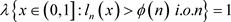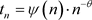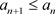# α-Lu¨ roth展式若干度量性质Some Metric Properties in α-Lu¨ roth Expansions

DOI: 10.12677/AAM.2020.91002, PDF, HTML, XML, 下载: 245  浏览: 1,945  科研立项经费支持

Abstract: For the α-Lüroth expansion, some metric properties, such as “0-1” law, iterated logarithm law of the digits are studied in this paper. As the extension of alternating-Lüroth expansion, the conclusions in this paper include those of alternating-Lüroth case.

1. 引言

${L}_{\alpha }\left(x\right):=\left\{\begin{array}{l}\frac{{t}_{n}-x}{{a}_{n}},\text{\hspace{0.17em}}\text{\hspace{0.17em}}x\in {A}_{n},n\in N\\ 0,\text{\hspace{0.17em}}\text{\hspace{0.17em}}\text{\hspace{0.17em}}\text{\hspace{0.17em}}\text{\hspace{0.17em}}\text{\hspace{0.17em}}\text{\hspace{0.17em}}\text{\hspace{0.17em}}\text{\hspace{0.17em}}\text{\hspace{0.17em}}\text{\hspace{0.17em}}\text{\hspace{0.17em}}x=0\end{array}$

$x={t}_{{l}_{1}\left(x\right)}+\underset{j=2}{\overset{\infty }{\sum }}{\left(-1\right)}^{j-1}\left(\underset{1\le i\le j}{\prod }{a}_{{l}_{1}\left(x\right)}\right){t}_{{l}_{j}\left(x\right)}$

1) 如果 $\underset{n=1}{\overset{\infty }{\sum }}\frac{\text{1}}{\varphi \left(n\right)}$ 发散，则 $\lambda \left(A\right)=0$,即2) 如果 $\underset{n=1}{\overset{\infty }{\sum }}\frac{\text{1}}{\varphi \left(n\right)}$ 收敛，则 $\lambda \left\{x\in \left(0,1\right]:{l}_{n}\left(x\right)>\varphi \left(n\right)\text{ }\text{\hspace{0.17em}}i.o.n\right\}=\text{0}$

${L}_{n}\left(x\right)=\mathrm{max}\left\{{l}_{1}\left(x\right),\cdots ,{l}_{n}\left(x\right)\right\}$

$\underset{n\to \infty }{\mathrm{lim}\mathrm{sup}}\frac{\mathrm{log}{l}_{n}\left(x\right)-\mathrm{log}n}{\mathrm{log}\mathrm{log}n}=1$

$\underset{n\to \infty }{\mathrm{lim}\mathrm{sup}}\frac{\mathrm{log}{L}_{n}\left(x\right)-\mathrm{log}n}{\mathrm{log}\mathrm{log}n}=\text{1}$

$\underset{n\to \infty }{\mathrm{lim}\mathrm{inf}}\frac{\mathrm{log}{l}_{n}\left(x\right)-\mathrm{log}n}{\mathrm{log}\mathrm{log}n}=-\infty$

$\underset{n\to \infty }{\mathrm{lim}\mathrm{inf}}\frac{\mathrm{log}{L}_{n}\left(x\right)-\mathrm{log}n}{\mathrm{log}\mathrm{log}n}=0$

2. 准备工作

$I\left({l}_{1},{l}_{2},\cdots ,{l}_{k}\right):=\left\{x\in \left[0,1\right):{l}_{i}\left(x\right)={l}_{i}\text{\hspace{0.17em}}\text{for}\text{\hspace{0.17em}}1\le i\le k\right\}$

1) 若对于 $\rho >1$，有 $\underset{n\to \infty }{\mathrm{lim}}\frac{{t}_{n}}{{t}_{n+1}}=\rho$ ；则 $\alpha$ 是扩张的；

2) 如果分割 $\alpha$ 的尾部满足幂律，，其中 $\psi :N\to {R}^{+}$ 是一个缓变函数，则 $\alpha$ 是指数 $\theta >0$ 的指数扩张的；

3) 如果n充分大，我们有，则分割 $\alpha$ 最终是递减的。

$\lambda \left(I\left({l}_{1},{l}_{2},\cdots ,{l}_{k}\right)\right)=\underset{i=1}{\overset{k}{\prod }}\lambda \left(I\left({l}_{i}\right)\right)$

${n}^{-\left(1+\theta +\eta \right)}\le {a}_{n}\le {n}^{-\left(1+\theta -\eta \right)}$(2.1)

${n}^{-\left(\theta +\eta \right)}\le {t}_{n}\le {n}^{-\left(\theta -\eta \right)}$(2.2)

$\underset{n\to \infty }{\mathrm{lim}}\frac{\mathrm{log}{a}_{n}}{n}=\underset{n\to \infty }{\mathrm{lim}}\frac{{t}_{n}}{{t}_{n+1}}=-\mathrm{log}\rho$

3. 主要结果的证明

3.1. 定理1.2的证明

${a}_{n}=\left\{\begin{array}{l}{n}_{k}\mathrm{log}{n}_{k}\text{\hspace{0.17em}}\text{\hspace{0.17em}}\text{\hspace{0.17em}}\text{\hspace{0.17em}}k\in N,n={n}_{k}\\ 0\text{\hspace{0.17em}}\text{\hspace{0.17em}}\text{\hspace{0.17em}}\text{\hspace{0.17em}}\text{\hspace{0.17em}}\text{\hspace{0.17em}}\text{\hspace{0.17em}}\text{\hspace{0.17em}}\text{\hspace{0.17em}}\text{\hspace{0.17em}}\text{\hspace{0.17em}}\text{\hspace{0.17em}}\text{\hspace{0.17em}}\text{\hspace{0.17em}}\text{\hspace{0.17em}}\text{\hspace{0.17em}}n\ne {n}_{k}\end{array}$

$\lambda \left\{x\in \left(0,1\right]:{l}_{n}\left(x\right)\le {a}_{n}\text{\hspace{0.17em}}i.o.n\right\}=1$

$\frac{\mathrm{log}{l}_{n}-\mathrm{log}n}{\mathrm{log}\mathrm{log}n}\le \frac{\mathrm{log}{a}_{n}-\mathrm{log}n}{\mathrm{log}\mathrm{log}n}$

$\underset{n\to \infty }{\mathrm{lim}\mathrm{sup}}\frac{\mathrm{log}{l}_{n}-\mathrm{log}n}{\mathrm{log}\mathrm{log}n}\le 1$

$\underset{n\to \infty }{\mathrm{lim}\mathrm{sup}}\frac{\mathrm{log}{l}_{n}-\mathrm{log}n}{\mathrm{log}\mathrm{log}n}=1$

$\underset{n\to \infty }{\mathrm{lim}\mathrm{sup}}\frac{\mathrm{log}{L}_{n}-\mathrm{log}n}{\mathrm{log}\mathrm{log}n}=1$

3.2. 定理1.3的证明

$\underset{n\to \infty }{\mathrm{lim}\mathrm{inf}}\frac{\mathrm{log}{l}_{n}-\mathrm{log}n}{\mathrm{log}\mathrm{log}n}\le -\infty$

$\theta \in \left(1,+\infty \right)$ 时，证明过程是相同的。然后，我们给出 ${L}_{n}$ 的“0-1”比率。

${b}_{n}=\left\{\begin{array}{l}{n}_{k}{\left(\mathrm{log}{n}_{k}\right)}^{\gamma }\text{\hspace{0.17em}}\text{\hspace{0.17em}}\text{\hspace{0.17em}}k\in N,n={n}_{k}\\ 0\text{\hspace{0.17em}}\text{\hspace{0.17em}}\text{\hspace{0.17em}}\text{\hspace{0.17em}}\text{\hspace{0.17em}}\text{\hspace{0.17em}}\text{\hspace{0.17em}}\text{\hspace{0.17em}}\text{\hspace{0.17em}}\text{\hspace{0.17em}}\text{\hspace{0.17em}}\text{\hspace{0.17em}}\text{\hspace{0.17em}}\text{\hspace{0.17em}}\text{\hspace{0.17em}}\text{\hspace{0.17em}}\text{\hspace{0.17em}}\text{\hspace{0.17em}}\text{\hspace{0.17em}}\text{\hspace{0.17em}}n\ne {n}_{k}\end{array}$

${\left(1-\frac{1}{{\left(n{\left(\mathrm{log}n\right)}^{\gamma }\right)}^{\left(\theta -\epsilon \right)}}\right)}^{n}\le \lambda \left({A}_{\gamma }\right)\le {\left(1-\frac{1}{{\left(n{\left(\mathrm{log}n\right)}^{\gamma }\right)}^{\left(\theta +\epsilon \right)}}\right)}^{n}$

$\begin{array}{l}\lambda \left\{\underset{m\ge 1}{\cap }\underset{n\ge m}{\cup }x\in \left[0,1\right):{L}_{n}\left(x\right)\le n{\left(\mathrm{log}n\right)}^{\gamma }\right\}\\ \ge \underset{n\to \infty }{\mathrm{lim}\mathrm{sup}}\lambda \left\{x\in \left[0,1\right):{L}_{n}\left(x\right)\le n{\left(\mathrm{log}n\right)}^{\gamma }\right\}\\ \ge \underset{n\to \infty }{\mathrm{lim}\mathrm{sup}}{\left(1-\frac{1}{{\left(n{\left(\mathrm{log}n\right)}^{\gamma }\right)}^{\left(\theta -\epsilon \right)}}\right)}^{n}\end{array}$

$\underset{n\to \infty }{\mathrm{lim}\mathrm{sup}}{\left(1-\frac{1}{{\left(n{\left(\mathrm{log}n\right)}^{\gamma }\right)}^{\left(\theta -\epsilon \right)}}\right)}^{n}\ge \underset{n\to \infty }{\mathrm{lim}}{\left(1-\frac{1}{{\left({n}^{\left[\frac{\text{2}}{\theta }\right]}{\left(\left[\frac{\text{2}}{\theta }\right]\mathrm{log}n\right)}^{\gamma }\right)}^{\left(\theta -\epsilon \right)}}\right)}^{n}\ge \underset{n\to \infty }{\mathrm{lim}}{\left(1-\frac{1}{{\left({n}^{\frac{\text{2}}{\theta }}{\left(\frac{\text{2}}{\theta }\mathrm{log}n\right)}^{\gamma }\right)}^{\left(\theta -\epsilon \right)}}\right)}^{n-p\left(n\right)}=1$

$\underset{n\to \infty }{\mathrm{lim}\mathrm{inf}}\frac{\mathrm{log}{L}_{n}-\mathrm{log}n}{\mathrm{log}\mathrm{log}n}\le \gamma$

$\underset{n\to \infty }{\mathrm{lim}\mathrm{inf}}\frac{\mathrm{log}{L}_{n}-\mathrm{log}n}{\mathrm{log}\mathrm{log}n}\le 0$

$\stackrel{¯}{{A}_{\frac{\tau }{\theta }}}=\left\{x\in \left[0,1\right):{L}_{n}\left(x\right)\le {n}^{{}_{\frac{1}{\theta }}}{\left(\mathrm{log}n\right)}^{-\frac{\tau }{\theta }},{L}_{n+1}\left(x\right)\le {\left(n+1\right)}^{{}^{{}_{\frac{1}{\theta }}}}{\left(\mathrm{log}\left(n+1\right)\right)}^{-\frac{\tau }{\theta }}\right\}$

$\begin{array}{c}\lambda \left(\stackrel{¯}{{A}_{\frac{\tau }{\theta }}}\right)=\lambda \left\{x\in \left[0,1\right):{L}_{n}\left(x\right)\le {n}^{{}_{\frac{1}{\theta }}}{\left(\mathrm{log}n\right)}^{-\frac{\tau }{\theta }},{L}_{n+1}\left(x\right)\le {\left(n+1\right)}^{{}^{{}_{\frac{1}{\theta }}}}{\left(\mathrm{log}\left(n+1\right)\right)}^{-\frac{\tau }{\theta }}\right\}\\ \le {\left(1-\frac{1}{{\left({n}^{\frac{\theta +\epsilon }{\theta }}{\left(\mathrm{log}n\right)}^{\gamma }\right)}^{-\frac{\tau \left(\theta +\epsilon \right)}{\theta }}}\right)}^{n}\frac{1}{{\left(n+1\right)}^{\frac{\theta -\epsilon }{\theta }}{\left(\mathrm{log}\left(n+1\right)\right)}^{-\frac{\tau \left(\theta +\epsilon \right)}{\theta }}}\\ \le {\text{e}}^{-\frac{1}{{\left(\mathrm{log}n\right)}^{-\tau }}}\cdot \frac{{\left(\mathrm{log}n\right)}^{\tau }}{n}\\ \le {\text{e}}^{-\frac{1}{{\left(\mathrm{log}n\right)}^{\tau }}}\cdot {n}^{\tau -1}\\ \le {\text{e}}^{-\frac{1}{{n}^{\tau }}}\cdot {n}^{\tau -1}\end{array}$

${\text{e}}^{-\frac{1}{{n}^{\tau }}}\cdot {n}^{\tau -1}=\underset{k=1}{\overset{\infty }{\sum }}{\left(-1\right)}^{k}\frac{1}{k!{n}^{k\tau }}\cdot {n}^{\tau -1}=\underset{k=1}{\overset{\infty }{\sum }}{\left(-1\right)}^{k}\frac{1}{k!{n}^{\left(k-1\right)\tau +1}}$

$\underset{n=1}{\overset{\infty }{\sum }}\lambda \left(\stackrel{¯}{{A}_{\frac{\tau }{\theta }}}\right)=\underset{n=1}{\overset{\infty }{\sum }}\frac{1}{{k}_{0}!{n}^{\left({k}_{0}-1\right)\tau }}\le +\infty$

$\lambda \left\{{L}_{n}\left(x\right)\le {n}^{\frac{1}{\theta }}{\left(\mathrm{log}n\right)}^{-\frac{\tau }{\theta }}\text{\hspace{0.17em}}\text{ }i.o.n\right\}=0$

$\underset{n\to \infty }{\mathrm{lim}\mathrm{inf}}\frac{\mathrm{log}{L}_{n}-\mathrm{log}n}{\mathrm{log}\mathrm{log}n}\ge -\frac{\tau }{\theta }$

$\underset{n\to \infty }{\mathrm{lim}\mathrm{inf}}\frac{\mathrm{log}{L}_{n}-\mathrm{log}n}{\mathrm{log}\mathrm{log}n}\ge -\text{0}$

  Munday, S. (2012) Finite and Infinite Ergodic Theory for Linear and Conformal Dynamical System. University of St. Andrews, UK.  Galambos, J. (1974) An Iterated Logarithm Type Theorem for the Largest Coefficient in Continued Fractions. Acta Arithmetica, 25, 359-364. https://doi.org/10.4064/aa-25-4-359-364  Khintchine A. Ya (1964) Continued Fractions. Chicago University Press, Chicago.  Kalpazidou, S., Knopfmacher, A. and Knopfmacher, J. (1991) Metric Properties of Alternating Lüroth Series. Portugaliae Mathematica, 48, 319-325.  Schweiger, F. (1995) Ergodic Theory of Fibred Systems and Metirc Number Theory. Clarendon Press, Oxford.  Dajani, K. and Kraaikamp, C. (1996) On Approximation by Lüroth Series. Journal de Théorie des Nombres de Bordeaux, 8, 331-346. https://doi.org/10.5802/jtnb.172  Oppenheim, A. (1972) The Representation of Real Numbers by Infinite Series of Rationals. Acta Arithmetica, 21, 391-398. https://doi.org/10.4064/aa-21-1-391-398  Kesseböhmer, K., Mundays, S. and Stratmann, B.O. (2012) Strong Renewal Theorems and Lyapunov Spectra for α-Farey and α-Lüroth Systems. Ergodic Theory and Dynamical Systems, 32, 989-1017. https://doi.org/10.1017/S0143385711000186